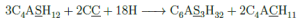# How to type a bar over/under a letter in mhchem of LaTeX?

I use usepackage mhchem to write chemical equations in LaTeX, it works fine most of time. Today I encountered a problem, how to type a horizontal bar over (or under) a letter?

After some Googling work, here is my solution, combining the command mathrm and overline/underline. The command \underline (\overline) is placing a bar under (over) a letter, while mathrm is remove the italic style of the letter in the math environment.

E.g. to get an chemical equation as follows:You can use the code below:

\begin{equation}
\ce{3 C4A\mathrm{\underline{S}}H12 + 2 C\mathrm{\underline{C}} + 18 H -> C6A$\mathrm{\underline{S}_3}$H32 + 2 C4A\mathrm{\underline{C}}H11}
\label{some label}
\end{equation}


You are welcome to share your trick with me, thanks.